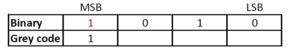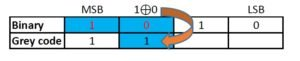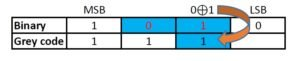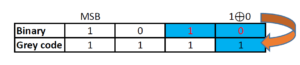Use the below online conversion tool to convert any decimal number to gray code:

 Decimal Number Gray Code

Also Try:

## Decimal Number

Whole number, a decimal point and a fractional value combines to form a decimal number. The decimal point separates the whole number part from the fractional part of the number. Each digit of a decimal number can be any number from 0 to 9. Any value less than 1 is written to the right of decimal point. Decimal numbers are also known as base-10 number or counting numbers. Place value of decimal number varies as the whole number powers of 10 starting from the left of decimal point. Similarly, the place value of digits left to decimal point varies as the division of power of tens.

## Gray code

Gray code, also known as reflected binary code, is a code having digits 0 and 1. Gray code do not have place value for its digits. Any successive codes in Gray code system have only one bit changes.

## Example for decimal to gray code conversion

Let us convert 1510 to gray code:
Step 1: The MSB (Most Significant Bit) of a gray code and binary code will be the same.Step 2: The next digit of gray code will be the EXOR of the MSB and the digit right to the MSB of the binary code.Step 3: Similarly EXOR the digit in place and the previous digit of binary code to obtain the next digit of gray code.Step 4: Repeat the previous step till the LSB of gray code is found.To convert decimal number to gray code, first we have to convert the number to binary.
1510 = 11112
Now, we can convert this binary number to gray code as follows

## Decimal to gray conversion table

Decimal NumberBinary NumberGray code
000000000
100010001
200100011
300110010
401000110
501010111
601100101
701110100
810001100
910011101
1010101111
1110111110
1211001010
1311011011
1411101001
1511111000

Also Try: